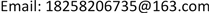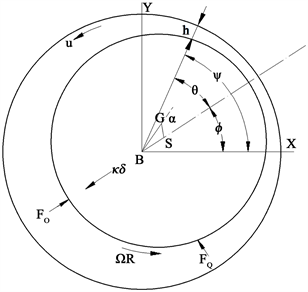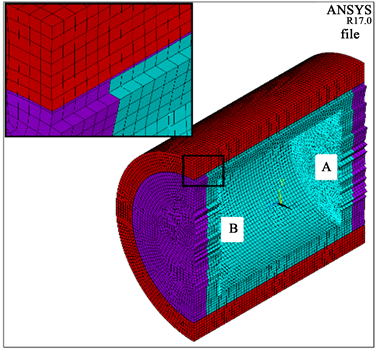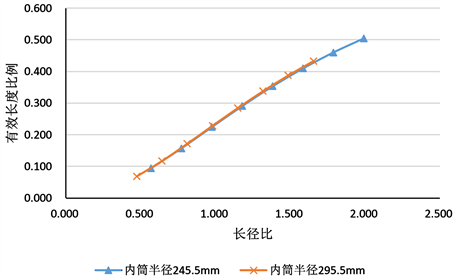﻿ 屏蔽泵电机定转子环域水对转子振动的影响 The Effect of an Annular Fluid on the Vibrations of a Canned Motor Pump’s Rotor

International Journal of Mechanics Research
Vol. 08  No. 02 ( 2019 ), Article ID: 30819 , 7 pages
10.12677/IJM.2019.82017

The Effect of an Annular Fluid on the Vibrations of a Canned Motor Pump’s Rotor

Fang Liu1,2, Yanpeng Bu1, Peng Wu1,2, Xufeng Wang1,2, Genfang Yu1,2

1Zhefu Holding Group Co., Ltd., Hangzhou Zhejiang

2Zhejiang Fuchanjiang Hydropower Equipment Co., Ltd., Hangzhou ZhejiangReceived: May 28th, 2019; accepted: Jun. 11th, 2019; published: Jun. 18th, 2019ABSTRACT

In the canned motor pump, fluid is contained in the thin annular regions between the rotor and stator in locations such as the can annulus and in seals. When relative motion takes place between rotor and stator, the constrained motion of the fluid can give rise to significant interaction effects. In a paper authored by R. J. Fritz, the effects of an annular fluid on the vibrations of a long rotor are investigated. The hydraulic mass term mH as defined in Fritz’s equations, is based on the assumption that the annular region is sufficiently long that end leakage effects are negligible. For this case, the length L is simply the actual length of fluid annulus being considered. But the rotor is always finite length; the influence of the length needs to be considered in the formula of mH. Therefore, the effects of fluid leakage at the end of the annular region can be taken into account by modifying the length L to get the effect length Leff.

Keywords:Canned Motor Pump, Fluid in the Thin Annular Regions, FRITZ, Hydro Mass, Effect Length

1浙富控股集团股份有限公司，浙江 杭州

2浙江富春江水电设备有限公司，浙江 杭州Copyright © 2019 by author(s) and Hans Publishers Inc.1. 简介

2. FRITZ基本理论(B、S为非转动体和转动体的中心；G为转动体质量中心；GS = e；BG = r；BS = $\delta$$\varphi$ 为极坐标系相对最小液膜厚度位置； $\psi$ 为环域某水体位置； $\theta$ 为环域某水体相对于最小液膜厚度的位置； $\Omega$ 为转动部件角速度)

Figure 1. The section of the annular

$\frac{\partial h}{\partial t}+\frac{1}{R}\frac{\partial hu}{\partial \Psi }=0$ (1)

$-\frac{1}{R}\frac{\partial p}{\partial \phi }=\frac{{\tau }_{1}-{\tau }_{2}}{h}+\rho \left(\frac{\partial u}{\partial t}+\frac{u}{R}\frac{\partial u}{\partial \phi }\right)$ (2)

${\tau }_{1},{\tau }_{2}$ ——水壁面的剪切应力

$\rho$ ——水体的密度

$\frac{{\tau }_{1}-{\tau }_{2}}{h}={F}_{V}\rho {\left(\frac{C}{h}\right)}^{1.217}V$ (3)

$\frac{{\tau }_{1}-{\tau }_{2}}{h}={F}_{T}\rho \left(\frac{C}{h}\right)V$ (4)

${F}_{V}$${F}_{T}$ 、F为阻尼系数

$-\frac{1}{R}\frac{\partial p}{\partial \phi }=\rho {\left(\frac{C}{h}\right)}^{a}FV+\rho \left(\frac{\partial u}{\partial t}+\frac{u}{R}\frac{\partial u}{\partial \phi }\right)$ (5)

$h=C\left(1-ϵ\mathrm{cos}\theta \right)$ (6)

$\psi =\theta \left(\psi ,t\right)+\varphi \left(t\right)$ (7)

$u=\frac{\Omega R}{2}\left(1+ϵ\mathrm{cos}\theta \right)+R\stackrel{˙}{ϵ}\mathrm{sin}\theta -Rϵ\stackrel{˙}{\varphi }\mathrm{cos}\theta$ (8)

$u=\frac{\Omega R}{2}+V$ (9)

$V=\frac{\Omega R}{2}ϵ\mathrm{cos}\theta +R\stackrel{˙}{ϵ}\mathrm{sin}\theta -Rϵ\stackrel{˙}{\varphi }\mathrm{cos}\theta$ (10)

$\partial \psi =\partial \theta \left(\partial t=0\right)$ (11)

$\begin{array}{c}-\frac{1}{\rho R}\frac{\partial p}{\partial \theta }=\mathrm{cos}\theta \left(-\Omega R\stackrel{˙}{ϵ}+2R\stackrel{˙}{ϵ}\stackrel{˙}{\varphi }+Rϵ\stackrel{¨}{\varphi }-\frac{\Omega R}{2}ϵF+Rϵ\stackrel{˙}{\varphi }F\right)\\ \text{\hspace{0.17em}}\text{\hspace{0.17em}}+\mathrm{sin}\theta \left(-\frac{\Omega R}{2}ϵ\stackrel{˙}{\varphi }-R\stackrel{¨}{ϵ}+Rϵ{\stackrel{˙}{\varphi }}^{2}+\frac{{\Omega }^{2}}{4}Rϵ-\frac{\Omega }{2}Rϵ\stackrel{˙}{\varphi }-R\stackrel{˙}{ϵ}F\right)\end{array}$ (12)

${F}_{0}=-{\int }_{0}^{\text{2π}}LRp\mathrm{cos}\theta \text{d}\theta$ (13)

${F}_{Q}=-{\int }_{0}^{\text{2π}}LRp\mathrm{sin}\theta \text{d}\theta$ (14)

$-{F}_{0}={m}_{H}C\left(\stackrel{¨}{ϵ}-ϵ{\stackrel{˙}{\varphi }}^{2}+\Omega ϵ\stackrel{˙}{\varphi }-\frac{{\Omega }^{2}}{4}ϵ+F\stackrel{˙}{E}\right)$

$-{F}_{Q}={m}_{H}C\left(ϵ\stackrel{¨}{\varphi }+2\stackrel{˙}{ϵ}\stackrel{˙}{\varphi }-\Omega \stackrel{˙}{ϵ}-\frac{\Omega }{2}ϵF+Fϵ\stackrel{˙}{\varphi }\right)$

3. 分析方法

FRITZ模型简化基于环域水与长转子半径比值远远大于1， $L/R\gg 1$ ；环域被认为是很薄的， $h\ll R$ 。而本文使用的模型对转子的长度没有限制，但仍针对很薄的环域 $h\ll R$

${m}_{H}=\text{π}\rho {R}^{3}{L}_{有效}/C$

$\eta ={L}_{有效}/L$

${L}_{有效}$ 为水动力质量的有效长度， $\eta$ 为有效长度比例。通过计算得到有效长度比例 随长径比变化的关系曲线。(长径比为内筒长度与直径的比值)。

4. 有限元分析模型Figure 2. The FEA modelTable 1. The geometric dimension of the FEA models

5. 分析结果Figure 3. The curved line between the ratio of effective length and the ratio of length to diameter of the annulusTable 2. The ratio of effective length to the ratio of length to diameter of the annulus

6. 结论

1) FRITZ公式基于环域水长度与转子直径比值远远大于1的情况， $L/R\gg 1$ ；这是较理想的状态，在很多实际分析中是不适用的；

2) 本文得到的环域水动力质量有效长度比例与长径比的关系曲线仅针对于水体薄环域， $h\ll R$ ，计算中没有考虑环域的阻尼和刚度；

3) 本文将两种常规较成熟的计算分析方法的计算结果进行对比，得出环域水动力质量有效长度比与长径比的关系曲线，对考虑水中模态的工程人员有一定借用价值；

4) 流固耦合与干模态虽为较成熟的计算方法，但有效长度关系曲线仍需试验进一步验证。

The Effect of an Annular Fluid on the Vibrations of a Canned Motor Pump’s Rotor[J]. 力学研究, 2019, 08(02): 149-155. https://doi.org/10.12677/IJM.2019.82017

1. 1. 成德. 大功率屏蔽式核主泵浸没转子系统动力学特性研究[D]: [博士学位论文]. 上海: 上海交通大学, 2016.

2. 2. 曲大庄, 金乐, 等. 间隙环流对核电主泵电机转子振动的影响[J]. 动力工程学报, 2015(35): pp.

3. 3. 蒋庆磊, 邢桂坤, 吴大转, 等. 离心泵内小间隙环流瞬态流体力计算[J]. 浙江大学学报, 2012, 46(5): 929-934.

4. 4. Fritz, R.J. (1970) The Effects of an Annular Fluid on the Vibrations of a Long Rotor, Part 1—Theory. Journal of Fluids Engineering, 92, 923-929. https://doi.org/10.1115/1.3425165

5. 5. Fritz, R.J. (1966) The Effects of an Annular Fluid on the Vibrations of a Long Rotor, Part 1—Test. Journal of Fluids Engineering, 150, 6-8.